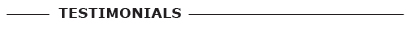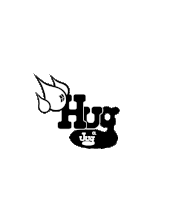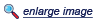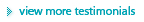The Water Project, Transform A LifeThis is a sample text area. This is a sample text area. This is a sample text area. This is a sample text area. This is a sample text area. This is a sample text area. This is a sample text area. This is a sample text area. This is a sample text area. This is a sample text area. This is a sample text area. This is a sample text area. This is a sample text area. This is a sample text area. This is a sample text area. This is a sample text area. This is a sample text area. This is a sample text area. This is a sample text area. This is a sample text area.This is a sample text area. This is a sample text area. This is a sample text area. This is a sample text area. This is a sample text area. This is a sample text area. This is a sample text area. This is a sample text area. This is a sample text area. This is a sample text area. This is a sample text area. This is a sample text area. This is a sample text area. This is a sample text area. This is a sample text area. This is a sample text area. This is a sample text area. This is a sample text area. This is a sample text area. This is a sample text area.
 This is a sample text area. This is a sample text area. This is a sample text area. This is a sample text area. This is a sample text area. This is a sample text area. This is a sample text area. This is a sample text area. This is a sample text area. This is a sample text area. This is a sample text area. This is a sample text area. This is a sample text area. This is a sample text area. This is a sample text area. This is a sample text area. This is a sample text area. This is a sample text area. This is a sample text area. This is a sample text area.